# Marine Corps 3451 Fiscal/Budget Technician ASVAB Study Guide

 Branch Marine Corps MOS 3451 Title Fiscal/Budget Technician Description Financial management resource analysts perform accounting, budgeting and all other financial management relevant duties for appropriated funds supporting the operating forces and supporting establishments. Financial management resource analyst perform duties specifically pertain to maintenance, monitoring, reconciliation and preparation of official accounting records; general ledger control; related cost accounting; financial systems analysis and any oversight duties typically performed by related cost accounting organizations.Construct a Line of Accounting (LOA); Construct a Document Number; Construct a Financial Information Pointer (FIP); Process Accounting Transactions into Standard Accounting Budgeting and Reporting System (SABRS); Maintain Local Tables in the Standard Accounting Budgeting and Reporting System (SABRS); Input Financial Data into PR Builder; Input Lines of Accounting into the Defense Travel System (DTS)Maintain Correspondence Files and Directives; Recommend Financial Management Policy/Guidance; Perform Budget Execution Analysis; Perform Fund Administrator Training; Reconcile Pre-validation Report; Validate Fiscal Year Closeout; Conduct Financial Management Sustainment Training Subtests Arithmetic Reasoning, Math Knowledge, Paragraph Comprehension, Word Knowledge

# Arithmetic Reasoning

• 13 Questions
• 54 Problems
• 36 Flash Cards

### Fundamentals

##### Number Properties
###### Integers

An integer is any whole number, including zero. An integer can be either positive or negative. Examples include -77, -1, 0, 55, 119.

###### Rational Numbers

A rational number (or fraction) is represented as a ratio between two integers, a and b, and has the form $${a \over b}$$ where a is the numerator and b is the denominator. An improper fraction ($${5 \over 3}$$) has a numerator with a greater absolute value than the denominator and can be converted into a mixed number ($$1 {2 \over 3}$$) which has a whole number part and a fractional part.

###### Absolute Value

The absolute value is the positive magnitude of a particular number or variable and is indicated by two vertical lines: $$\left|-5\right| = 5$$. In the case of a variable absolute value ($$\left|a\right| = 5$$) the value of a can be either positive or negative (a = -5 or a = 5).

###### Factors & Multiples

A factor is a positive integer that divides evenly into a given number. The factors of 8 are 1, 2, 4, and 8. A multiple is a number that is the product of that number and an integer. The multiples of 8 are 0, 8, 16, 24, ...

###### Greatest Common Factor

The greatest common factor (GCF) is the greatest factor that divides two integers.

###### Least Common Multiple

The least common multiple (LCM) is the smallest positive integer that is a multiple of two or more integers.

###### Prime Number

A prime number is an integer greater than 1 that has no factors other than 1 and itself. Examples of prime numbers include 2, 3, 5, 7, and 11.

##### Operations on Fractions
###### Simplifying Fractions

Fractions are generally presented with the numerator and denominator as small as is possible meaning there is no number, except one, that can be divided evenly into both the numerator and the denominator. To reduce a fraction to lowest terms, divide the numerator and denominator by their greatest common factor (GCF).

Fractions must share a common denominator in order to be added or subtracted. The common denominator is the least common multiple of all the denominators.

###### Multiplying & Dividing Fractions

To multiply fractions, multiply the numerators together and then multiply the denominators together. To divide fractions, invert the second fraction (get the reciprocal) and multiply it by the first.

##### Operations on Exponents
###### Defining Exponents

An exponent (cbe) consists of coefficient (c) and a base (b) raised to a power (e). The exponent indicates the number of times that the base is multiplied by itself. A base with an exponent of 1 equals the base (b1 = b) and a base with an exponent of 0 equals 1 ( (b0 = 1).

To add or subtract terms with exponents, both the base and the exponent must be the same. If the base and the exponent are the same, add or subtract the coefficients and retain the base and exponent. For example, 3x2 + 2x2 = 5x2 and 3x2 - 2x2 = x2 but x2 + x4 and x4 - x2 cannot be combined.

###### Multiplying & Dividing Exponents

To multiply terms with the same base, multiply the coefficients and add the exponents. To divide terms with the same base, divide the coefficients and subtract the exponents. For example, 3x2 x 2x2 = 6x4 and $${8x^5 \over 4x^2}$$ = 2x(5-2) = 2x3.

###### Exponent to a Power

To raise a term with an exponent to another exponent, retain the base and multiply the exponents: (x2)3 = x(2x3) = x6

###### Negative Exponent

A negative exponent indicates the number of times that the base is divided by itself. To convert a negative exponent to a positive exponent, calculate the positive exponent then take the reciprocal: $$b^{-e} = { 1 \over b^e }$$. For example, $$3^{-2} = {1 \over 3^2} = {1 \over 9}$$

Radicals (or roots) are the opposite operation of applying exponents. With exponents, you're multiplying a base by itself some number of times while with roots you're dividing the base by itself some number of times. A radical term looks like $$\sqrt[d]{r}$$ and consists of a radicand (r) and a degree (d). The degree is the number of times the radicand is divided by itself. If no degree is specified, the degree defaults to 2 (a square root).

The radicand of a simplified radical has no perfect square factors. A perfect square is the product of a number multiplied by itself (squared). To simplify a radical, factor out the perfect squares by recognizing that $$\sqrt{a^2} = a$$. For example, $$\sqrt{64} = \sqrt{16 \times 4} = \sqrt{4^2 \times 2^2} = 4 \times 2 = 8$$.

To add or subtract radicals, the degree and radicand must be the same. For example, $$2\sqrt{3} + 3\sqrt{3} = 5\sqrt{3}$$ but $$2\sqrt{2} + 2\sqrt{3}$$ cannot be added because they have different radicands.

To multiply or divide radicals, multiply or divide the coefficients and radicands separately: $$x\sqrt{a} \times y\sqrt{b} = xy\sqrt{ab}$$ and $${x\sqrt{a} \over y\sqrt{b}} = {x \over y}\sqrt{a \over b}$$

###### Square Root of a Fraction

To take the square root of a fraction, break the fraction into two separate roots then calculate the square root of the numerator and denominator separately. For example, $$\sqrt{9 \over 16}$$ = $${\sqrt{9}} \over {\sqrt{16}}$$ = $${3 \over 4}$$

##### Miscellaneous
###### Scientific Notation

Scientific notation is a method of writing very small or very large numbers. The first part will be a number between one and ten (typically a decimal) and the second part will be a power of 10. For example, 98,760 in scientific notation is 9.876 x 104 with the 4 indicating the number of places the decimal point was moved to the left. A power of 10 with a negative exponent indicates that the decimal point was moved to the right. For example, 0.0123 in scientific notation is 1.23 x 10-2.

###### Factorials

A factorial has the form n! and is the product of the integer (n) and all the positive integers below it. For example, 5! = 5 x 4 x 3 x 2 x 1 = 120.

### Applications

##### Order of Operations
###### PEMDAS

Arithmetic operations must be performed in the following specific order:

1. Parentheses
2. Exponents
3. Multiplication and Division (from L to R)
4. Addition and Subtraction (from L to R)

The acronym PEMDAS can help remind you of the order.

###### Distributive Property - Multiplication

The distributive property for multiplication helps in solving expressions like a(b + c). It specifies that the result of multiplying one number by the sum or difference of two numbers can be obtained by multiplying each number individually and then totaling the results: a(b + c) = ab + ac. For example, 4(10-5) = (4 x 10) - (4 x 5) = 40 - 20 = 20.

###### Distributive Property - Division

The distributive property for division helps in solving expressions like $${b + c \over a}$$. It specifies that the result of dividing a fraction with multiple terms in the numerator and one term in the denominator can be obtained by dividing each term individually and then totaling the results: $${b + c \over a} = {b \over a} + {c \over a}$$. For example, $${a^3 + 6a^2 \over a^2} = {a^3 \over a^2} + {6a^2 \over a^2} = a + 6$$.

###### Commutative Property

The commutative property states that, when adding or multiplying numbers, the order in which they're added or multiplied does not matter. For example, 3 + 4 and 4 + 3 give the same result, as do 3 x 4 and 4 x 3.

##### Ratios
###### Ratios

Ratios relate one quantity to another and are presented using a colon or as a fraction. For example, 2:3 or $${2 \over 3}$$ would be the ratio of red to green marbles if a jar contained two red marbles for every three green marbles.

###### Proportions

A proportion is a statement that two ratios are equal: a:b = c:d, $${a \over b} = {c \over d}$$. To solve proportions with a variable term, cross-multiply: $${a \over 8} = {3 \over 6}$$, 6a = 24, a = 4.

###### Rates

A rate is a ratio that compares two related quantities. Common rates are speed = $${distance \over time}$$, flow = $${amount \over time}$$, and defect = $${errors \over units}$$.

###### Percentages

Percentages are ratios of an amount compared to 100. The percent change of an old to new value is equal to 100% x $${ new - old \over old }$$.

##### Statistics
###### Averages

The average (or mean) of a group of terms is the sum of the terms divided by the number of terms. Average = $${a_1 + a_2 + ... + a_n \over n}$$

###### Sequence

A sequence is a group of ordered numbers. An arithmetic sequence is a sequence in which each successive number is equal to the number before it plus some constant number.

###### Probability

Probability is the numerical likelihood that a specific outcome will occur. Probability = $${ \text{outcomes of interest} \over \text{possible outcomes}}$$. To find the probability that two events will occur, find the probability of each and multiply them together.

##### Word Problems
###### Practice

Many of the arithmetic reasoning problems on the ASVAB will be in the form of word problems that will test not only the concepts in this study guide but those in Math Knowledge as well. Practice these word problems to get comfortable with translating the text into math equations and then solving those equations.

# Math Knowledge

• 28 Questions
• 38 Problems
• 55 Flash Cards

### Algebra

##### Expressions
###### Classifications

A monomial contains one term, a binomial contains two terms, and a polynomial contains more than two terms. Linear expressions have no exponents. A quadratic expression contains variables that are squared (raised to the exponent of 2).

###### Operations Involving Monomials

You can only add or subtract monomials that have the same variable and the same exponent. However, you can multiply and divide monomials with unlike terms.

###### Multiplying Binomials

To multiply binomials, use the FOIL method. FOIL stands for First, Outside, Inside, Last and refers to the position of each term in the parentheses.

To factor a quadratic expression, apply the FOIL (First, Outside, Inside, Last) method in reverse.

##### Solving Equations
###### One Variable

An equation is two expressions separated by an equal sign. The key to solving equations is to repeatedly do the same thing to both sides of the equation until the variable is isolated on one side of the equal sign and the answer on the other.

###### Two Variables

When solving an equation with two variables, replace the variables with the values given and then solve the now variable-free equation. (Remember order of operations, PEMDAS, Parentheses, Exponents, Multiplication/Division, Addition/Subtraction.)

###### Two Equations

When presented with two equations with two variables, evaluate the first equation in terms of the variable you're not solving for then insert that value into the second equation. For example, if you have x and y as variables and you're solving for x, evaluate one equation in terms of y and insert that value into the second equation then solve it for x.

When solving quadratic equations, if the equation is not set equal to zero, first manipulate the equation so that it is set equal to zero: ax2 + bx + c = 0. Then, factor the quadratic and, because it's set to zero, you know that one of the factors must equal zero for the equation to equal zero. Finding the value that will make each factor, i.e. (x + ?), equal to zero will give you the possible value(s) of x.

###### Inequalities

Solving equations with an inequality (<, >) uses the same process as solving equations with an equal sign. Isolate the variable that you're solving for on one wide of the equation and put everything else on the other side. The only difference is that your answer will be expressed as an inequality (x > 5) and not as an equality (x = 5).

### Geometry

##### Lines & Angles
###### Line Segment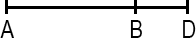A line segment is a portion of a line with a measurable length. The midpoint of a line segment is the point exactly halfway between the endpoints. The midpoint bisects (cuts in half) the line segment.

###### Right AngleA right angle measures 90 degrees and is the intersection of two perpendicular lines. In diagrams, a right angle is indicated by a small box completing a square with the perpendicular lines.

###### Acute & Obtuse Angles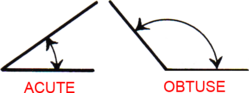An acute angle measures less than 90°. An obtuse angle measures more than 90°.

###### Angles Around Lines & Points

Angles around a line add up to 180°. Angles around a point add up to 360°. When two lines intersect, adjacent angles are supplementary (they add up to 180°) and angles across from either other are vertical (they're equal).

###### Parallel Lines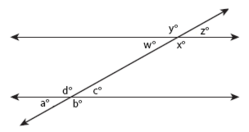Parallel lines are lines that share the same slope (steepness) and therefore never intersect. A transversal occurs when a set of parallel lines are crossed by another line. All of the angles formed by a transversal are called interior angles and angles in the same position on different parallel lines equal each other (a° = w°, b° = x°, c° = z°, d° = y°) and are called corresponding angles. Alternate interior angles are equal (a° = z°, b° = y°, c° = w°, d° = x°) and all acute angles (a° = c° = w° = z°) and all obtuse angles (b° = d° = x° = y°) equal each other. Same-side interior angles are supplementary and add up to 180° (e.g. a° + d° = 180°, d° + c° = 180°).

##### Polygons
###### Triangle Geometry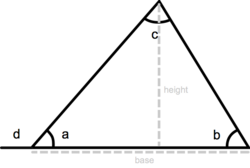A triangle is a three-sided polygon. It has three interior angles that add up to 180° (a + b + c = 180°). An exterior angle of a triangle is equal to the sum of the two interior angles that are opposite (d = b + c). The perimeter of a triangle is equal to the sum of the lengths of its three sides, the height of a triangle is equal to the length from the base to the opposite vertex (angle) and the area equals one-half triangle base x height: a = ½ base x height.

###### Triangle Classification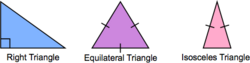An isosceles triangle has two sides of equal length. An equilateral triangle has three sides of equal length. In a right triangle, two sides meet at a right angle.

###### Pythagorean Theorem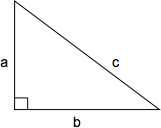The Pythagorean theorem defines the relationship between the side lengths of a right triangle. The length of the hypotenuse squared (c2) is equal to the sum of the two perpendicular sides squared (a2 + b2): c2 = a2 + b2 or, solved for c, $$c = \sqrt{a + b}$$A quadrilateral is a shape with four sides. The perimeter of a quadrilateral is the sum of the lengths of its four sides (a + b + c + d).

###### Rectangle & Square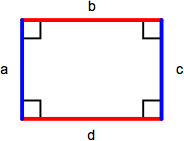A rectangle is a parallelogram containing four right angles. Opposite sides (a = c, b = d) are equal and the perimeter is the sum of the lengths of all sides (a + b + c + d) or, comonly, 2 x length x width. The area of a rectangle is length x width. A square is a rectangle with four equal length sides. The perimeter of a square is 4 x length of one side (4s) and the area is the length of one side squared (s2).

###### Parallelogram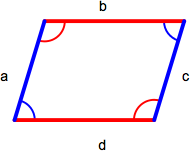A parallelogram is a quadrilateral with two sets of parallel sides. Opposite sides (a = c, b = d) and angles (red = red, blue = blue) are equal. The area of a parallelogram is base x height and the perimeter is the sum of the lengths of all sides (a + b + c + d).

###### Rhombus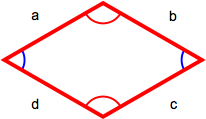A rhombus has four equal-length sides with opposite sides parallel to each other. The perimiter is the sum of the lengths of all sides (a + b + c + d) or, because all sides are the same length, 4 x length of one side (4s).

###### Trapezoid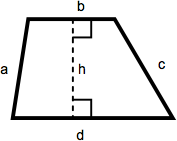A trapezoid is a quadrilateral with one set of parallel sides. The area of a trapezoid is one-half the sum of the lengths of the parallel sides multiplied by the height. In this diagram, that becomes ½(b + d)(h).

##### Circles
###### Dimensions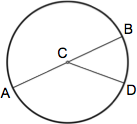A circle is a figure in which each point around its perimeter is an equal distance from the center. The radius of a circle is the distance between the center and any point along its perimeter (AC, CB, CD). A chord is a line segment that connects any two points along its perimeter (AB, AD, BD). The diameter of a circle is the length of a chord that passes through the center of the circle (AB) and equals twice the circle's radius (2r).

###### Calculations

The circumference of a circle is the distance around its perimeter and equals π (approx. 3.14159) x diameter: c = π d. The area of a circle is π x (radius)2 : a = π r2.

##### Solids
###### Cubes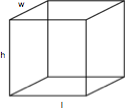A cube is a rectangular solid box with a height (h), length (l), and width (w). The volume is h x l x w and the surface area is 2lw x 2wh + 2lh.

###### Cylinders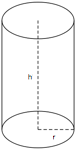A cylinder is a solid figure with straight parallel sides and a circular or oval cross section with a radius (r) and a height (h). The volume of a cylinder is π r2h and the surface area is 2(π r2) + 2π rh.

##### Coordinate Geometry
###### Coordinate Grid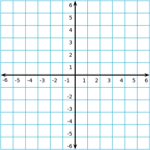The coordinate grid is composed of a horizontal x-axis and a vertical y-axis. The center of the grid, where the x-axis and y-axis meet, is called the origin.

###### Slope-Intercept Equation

A line on the coordinate grid can be defined by a slope-intercept equation: y = mx + b. For a given value of x, the value of y can be determined given the slope (m) and y-intercept (b) of the line. The slope of a line is change in y over change in x, $${\Delta y \over \Delta x}$$, and the y-intercept is the y-coordinate where the line crosses the vertical y-axis.